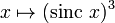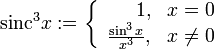# Sinc-cubed function$x \mapsto (\operatorname{sinc} \ x)^3$$\operatorname{sinc}^3 x := \left\lbrace \begin{array}{rl} 1, & x = 0 \\ \frac{\sin^3x}{x^3}, & x \ne 0 \\\end{array}\right.$